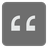Goes Right After
Elementary+
English

In a given word you need to check if one symbol goes right after another.

Cases you should expect while solving this challenge:

• If more than one symbol is in the list you should always count the first one
• one of the symbols are not in the given word - your function should return False;
• any symbol appears in a word more than once - use only the first one;
• two symbols are the same - your function should return False;
• the condition is case sensitive, which means 'a' and 'A' are two different symbols.

Input: Three arguments. The first one is a given string, second is a symbol that should go first, and the third is a symbold that should go after the first one.

Output: A bool.

Example:

```goes_after('world', 'w', 'o') == True
goes_after('world', 'w', 'r') == False
goes_after('world', 'l', 'o') == False
goes_after('panorama', 'a', 'n') == True
goes_after('list', 'l', 'o') == False
goes_after('', 'l', 'o') == False
goes_after('list', 'l', 'l') == False
goes_after('world', 'd', 'w') == False
```